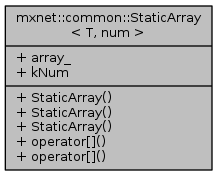This project has retired. For details please refer to its Attic page.
mxnet: mxnet::common::StaticArray< T, num > Struct Template Reference
 mxnet
mxnet::common::StaticArray< T, num > Struct Template Reference

Static array. This code is borrowed from struct Shape<ndim>, except that users can specify the type of the elements of the statically allocated array. The object instance of the struct is copyable between CPU and GPU. More...

`#include <static_array.h>`

Collaboration diagram for mxnet::common::StaticArray< T, num >:## Public Member Functions

default constructor, do nothing More...

constructor, fill in the array with the input value More...

MSHADOW_XINLINE StaticArray (const StaticArray< T, num > &sa)
constuctor More...

MSHADOW_XINLINE T & operator[] (const index_t idx)

MSHADOW_XINLINE const T & operator[] (const index_t idx) const

array_ [kNum]

## Static Public Attributes

static const int kNum = num

## Detailed Description

### template<typename T, int num> struct mxnet::common::StaticArray< T, num >

Static array. This code is borrowed from struct Shape<ndim>, except that users can specify the type of the elements of the statically allocated array. The object instance of the struct is copyable between CPU and GPU.

Template Parameters
 T element type of the array, must be copyable between CPU and GPU num number of elements in the array

## Constructor & Destructor Documentation

template<typename T, int num>
 MSHADOW_XINLINE mxnet::common::StaticArray< T, num >::StaticArray ( void )
inline

default constructor, do nothing

template<typename T, int num>
 MSHADOW_XINLINE mxnet::common::StaticArray< T, num >::StaticArray ( const T & val )
inline

constructor, fill in the array with the input value

template<typename T, int num>
 MSHADOW_XINLINE mxnet::common::StaticArray< T, num >::StaticArray ( const StaticArray< T, num > & sa )
inline

constuctor

## Member Function Documentation

template<typename T, int num>
 MSHADOW_XINLINE T& mxnet::common::StaticArray< T, num >::operator[] ( const index_t idx )
inline
template<typename T, int num>
 MSHADOW_XINLINE const T& mxnet::common::StaticArray< T, num >::operator[] ( const index_t idx ) const
inline

## Member Data Documentation

template<typename T, int num>
 T mxnet::common::StaticArray< T, num >::array_[kNum]
template<typename T, int num>
 const int mxnet::common::StaticArray< T, num >::kNum = num
static

The documentation for this struct was generated from the following file: